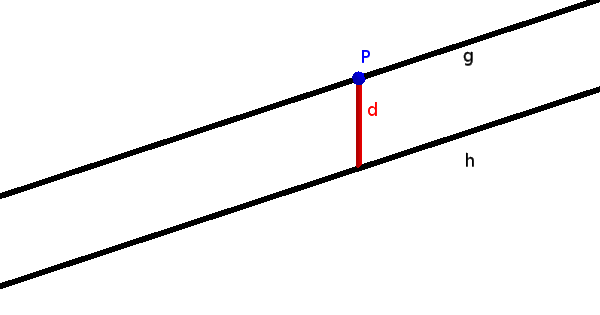Math Distance calculations Parallel lines

# Distance between parallel lines

Parallel lines have a constant distance everywhere.i

### Info

Intersecting and coincident lines have a distance of 0. Distance calculation is possible with skew and parallel lines.

Here, too, we can simply choose a point on a line and calculate the distance between a point and the straight line.

i

### Method

1. Check parallelism
2. Select point (support point)
3. Distance of the point to the other line

### Example

$\text{g: } \vec{x} = \begin{pmatrix} 1 \\ 2 \\ 1 \end{pmatrix} + r \cdot \begin{pmatrix} 1 \\ 1 \\ 0 \end{pmatrix}$

$\text{h: } \vec{x} = \begin{pmatrix} -1 \\ 0 \\ 3 \end{pmatrix} + r \cdot \begin{pmatrix} 3 \\ 3 \\ 0 \end{pmatrix}$

1. #### Check parallelism

Since this method only works with parallel lines, you have to check whether the lines are parallel.

We look at whether the direction vectors are collinear (parallel).

$\vec{a}=r\cdot\vec{b}$

$\begin{pmatrix} 1 \\ 1 \\ 0 \end{pmatrix}=r\cdot\begin{pmatrix}3 \\ 3 \\ 0 \end{pmatrix}$ $\Rightarrow r=3$

There is an $r$: The vectors are multiples of one another and therefore parallel.

2. #### Select point

You can take any point on the line. However, since you can easily read off the support point, this is a good option.

$P(-1|0|3)$

3. #### Distance of the point to the other line

The distance can now be calculated as described under distance point and line.

First set up an auxiliary plane with $P$ as the support point and direction vector as the normal vector.

$\text{H: } (\vec{x} - \vec{a}) \cdot \vec{n}=0$

$\text{H: } (\vec{x} - \begin{pmatrix} -1 \\ 0 \\ 3 \end{pmatrix}) \cdot \begin{pmatrix} 1 \\ 1 \\ 0 \end{pmatrix}=0$

The perpendicular point is the intersection of the line $g$ and the auxiliary plane $H$. To calculate the intersection, the equation of a line for $\vec{x}$ is used in the plane.

$\left(\color{red}{\begin{pmatrix} 1 \\ 2 \\ 1 \end{pmatrix} + r \cdot \begin{pmatrix} 1 \\ 1 \\ 0 \end{pmatrix}} - \begin{pmatrix} -1 \\ 0 \\ 3 \end{pmatrix}\right)$ $\cdot \begin{pmatrix} 1 \\ 1 \\ 0 \end{pmatrix}=0$

$\begin{pmatrix} 2+r \\ 2+r \\ -2 \end{pmatrix}$ $\cdot \begin{pmatrix} 1 \\ 1 \\ 0 \end{pmatrix}=0$

Calculate scalar product

$(2+r)\cdot1+(2+r)\cdot1$ $+(-2)\cdot0=0$
$4+2r=0\quad|-4$
$2r=-4\quad|:2$
$r=-2$

Insert $r$ in $g$ to get perpendicular point $F$.

$\vec{OF} = \begin{pmatrix} 1 \\ 2 \\ 1 \end{pmatrix} \color{red}{-2} \cdot \begin{pmatrix} 1 \\ 1 \\ 0 \end{pmatrix}$ $= \begin{pmatrix} 1-2 \\ 2-2 \\ 1-0 \end{pmatrix}$ $= \begin{pmatrix} -1 \\ 0 \\ 1 \end{pmatrix}$

$F(-1|0|1)$

The distance from the perpendicular point to point $P$ is also the distance from the line to this point.

The distance between two points can be easily calculated using vectors.

$d=|\vec{PF}|$ $=\left| \begin{pmatrix} -1-(-1) \\ 0-0 \\ 1-3 \end{pmatrix}\right|$ $=\left| \begin{pmatrix} 0 \\ 0 \\ -2 \end{pmatrix}\right|$ $=\sqrt{(-2)^2}$ $=2$

The distance between the two lines $g$ and $h$ is 2 LU.